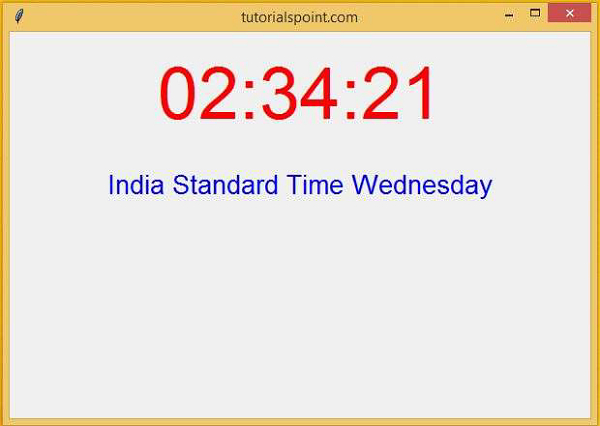# How do I create an automatically updating GUI using Tkinter?

PythonTkinterGUI-Programming

#### Beyond Basic Programming - Intermediate Python

Most Popular

36 Lectures 3 hours

#### Practical Machine Learning using Python

Best Seller

91 Lectures 23.5 hours

#### Practical Data Science using Python

22 Lectures 6 hours

Using Tkinter, we can create GUI which can be configured for auto-updation. For this purpose, we will create GUI-based clocks that will automatically get refreshed when the time changes. We will display the clock along with the day and TimeZone.

First, we will import the tkinter library in the notebook, then we will create a function which creates instances of current time and day using the “strftime” function.

## Example

#Import the tkinter library
from tkinter import *
import time
#Create an instance of the canvas
win = Tk()

#Select the title of the window
win.title("tutorialspoint.com")

#Define the geometry of the window
win.geometry("600x400")
#Define the clock which
def clock():
hh= time.strftime("%I")
mm= time.strftime("%M")
ss= time.strftime("%S")
day=time.strftime("%A")
ap=time.strftime("%p")
time_zone= time.strftime("%Z")
my_lab.config(text= hh + ":" + mm +":" + ss)
my_lab.after(1000,clock)

my_lab1.config(text=time_zone+" "+ day)
#Update the Time
def updateTime():
my_lab.config(text= "New Text")

#Creating the label with text property of the clock
my_lab= Label(win,text= "",font=("sans-serif", 56), fg= "red")
my_lab1= Label(win, text= "", font=("Helvetica",20), fg= "blue")

#Calling the clock function
clock()
#Keep Running the window

win.mainloop()

## Output

Running the above code will create an automatically updated GUI Application in the window.